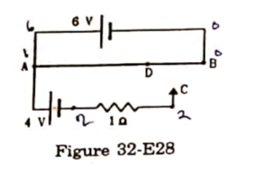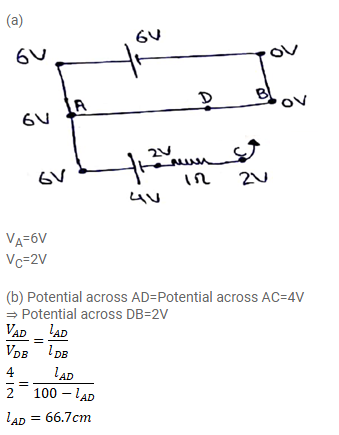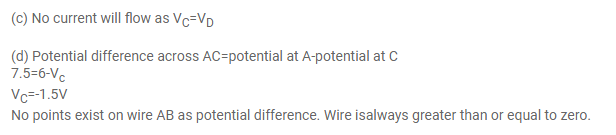# A 6 volt battery of negligible internal resistance is connected across a uniform

Question:

A 6 volt battery of negligible internal resistance is connected across a uniform wire AB of length $100 \mathrm{~cm}$. The positive terminal of another battery of emf $4 \mathrm{~V}$ and internal resistance $1 \Omega$ is joined to the point A as shown in the figure (32-E28). Take the potential at $B$ to be zero.

(a) What are the potentials at the points $A$ and $C$ ?

(b) At which point $D$ of the wire $A B$, the potential is equal to the potential at $C$ ?

(c) If the points $C$ and $D$ are connected by a wire, what will be the current through it?

(d) If the $4 \mathrm{~V}$ battery is replaced by $7.5 \mathrm{~V}$ battery, what would be the answers of parts (a) and (b)?Solution: